# Round Model Math

Our round modeling tool allows you to calculate the effect that taking on a new round of funding will have on your existing shareholders. To make it as usable as possible our interface hides many of the low level calculations – we're trying to make it easy for you – but we thought we'd explain the mathematics behind the scenes in case you wanted to run the calculation yourself.

We take only three inputs (plus your existing cap table!). The pre-money valuation of the company, the incoming investment and an optional post-money option pool. From these we can calculate everyone’s dilution and percentage ownership once the money comes in.

The model works in two stages:

1. We work out the effective pre-money (Eff-Pre) valuation of the company. This is most likely lower than the top line pre-money valuation given to you in your term sheet. Here we must broach the Option Pool Shuffle (there's no better explanation of this than Venture Hack's) and the impact of any convertible note instruments.
2. We use this Eff-Pre to calculate how many shares are bought, created and converted.

Note that whilst the new options are treated as part of the investment round, they are almost always (as we do here) factored in pre-money so they dilute existing shareholders not the incoming investors.

Throughout I’ll lay out the raw algebra but also follow through with an example (in italics).

We’ll use a company with just three securities:

• Common - An Equity Series with 90,000 shares issued
• Options - An options series with 10,000 authorized but only 5,000 issued
• Debt A - A Debt series with \$100,000 invested at a 20% discount.

This company is doing a Series A of \$1,000,000 at a pre-money valuation of \$4,000,000. The incoming investors are requesting a 10% post-money option pool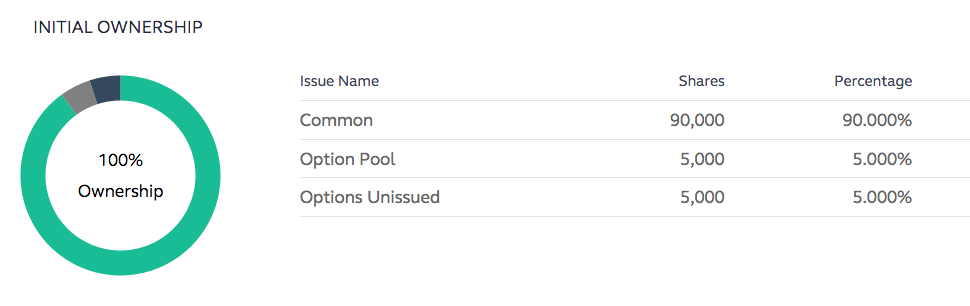## Note Impact

The value of the notes is fairly straightforward to calculate:

If there is no valuation cap, use the note's flat discount. Otherwise, the discount on the note is the larger of: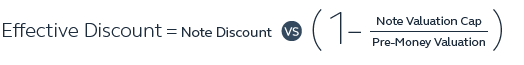This gives the note the following value:This has to be repeated for each note (though if they're all on the same terms you can calculate them all at once).

We have one \$100,000 note at a 20% discount. There’s no valuation cap so we take that discount as is and divide 100,000 by 0.8 giving us a note value of \$125,000.

## Post-money Option Pool Impact

Next we have to handle the option pool shuffle. The incoming investors want a post-money option pool of a specific percent (PostOPP). You already have an existing option pool, some of which is still unissued. This means you can roll your existing unissued options into the new pool.

First we calculate what percentage option pool size we need to have pre-money (PreOPP) to get the correct PostOPP: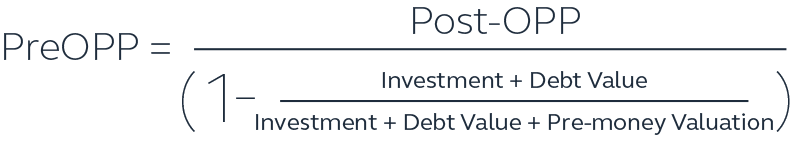Then factor in the existing unissued options, to reveal the percentage of options we have to create:Which then lowers the Eff-Pre: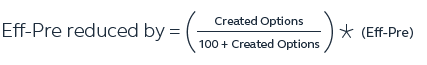You can simplify this hugely if you have no existing unissued options, but that’s not often the case in practice.

We already have 5% unissued options so we only have to boost this up to 10%. Using the first equation, it’s 10% after dilution so pre-money we have to increase it to 12.812% (our PreOPP). The increase to this amount lowers the Eff-Pre by \$328,947.37.

## Calculating Price-per-Share

We now have our Eff-Pre which also gives us a share price for our incoming investors (and note holders):The Eff-pre is then \$4,000,000 - \$328,947.37 which comes out at \$3,671,052.63 giving us a Price per share of \$36.71

## Calculating Ownership Percentage

With our final price per share calculated we now have everything we need to calculate the new ownership.

## Option Pool Increase

Creating the new option pool shares is essentially the same mathematics as the option pool impact above, only using shares rather than percentages.The options created bring the unissued options up from 5,000 to 13,961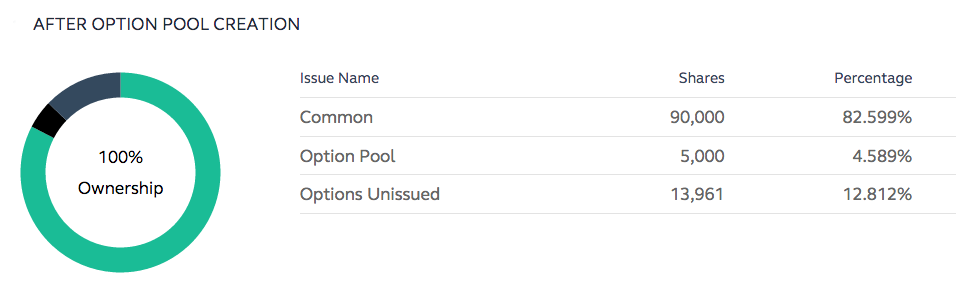## Note Conversion

For converting the notes we use the same effective discount calculated above for each note (the larger of the discount vs valuation cap discount)The \$100,000 note converts to 3,405 shares at a discounted price of 29.369.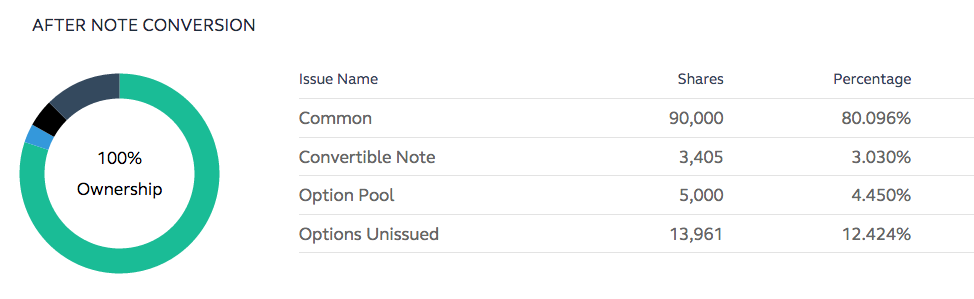New Series Shares

Finally we generate the number of shares that the investor gets for their money:The New Series holders get 27,240 shares for their \$1,000,000 investment and 19.512% of the company.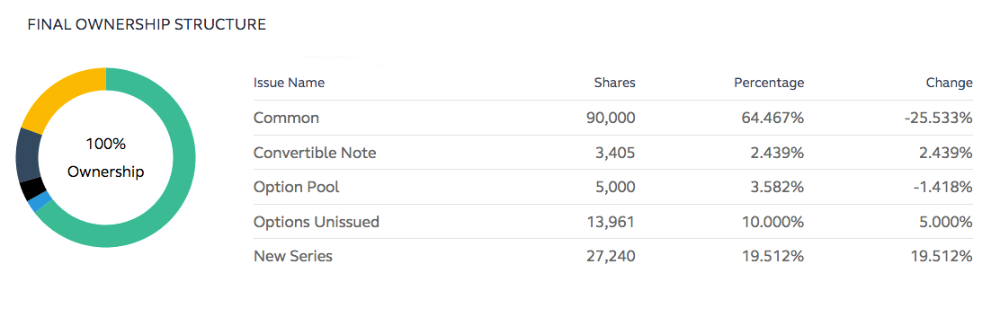Conclusion

You can check that all the above worked by multiplying the New Series Shares by our earlier calculated Price Per Share. If that sum doesn't equal the Investment Amount… something's gone wrong!

If you've got this far – bravo! It's confusing, not well understood mathematics, but it is of course massively important. I hope at this stage I've given you enough such that you could do it all yourself (though fortunately our tools will do it all for you!).